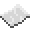# vector.sub

Given two vectors, self and object, this function will subtract object from self to create a new vector. This can also be written as `self - object`. If either argument was not created with vector.new, this function will not work as designed.ExampleSubtracts two vectors
Creates two new vectors then adds them together.
Code
```<nowiki>
local vectorA = vector.new(240, 71, -3040)
local vectorB = vector.new(2553, 32, -314)
local vectorC = vector.sub(vectorA, vectorB)
print("My new vector has the components:", vectorC.x, vectorC.y, vectorC.z)
</nowiki>
```
Output My new vector has the components: -2313 39 -2726

vector.sub
Function
Syntax
vector.sub(
• self : table
• object : table
)
Colon notation: self:sub(
• object : table
)

Returns table vector
API vector
Source CC:Tweaked (source)